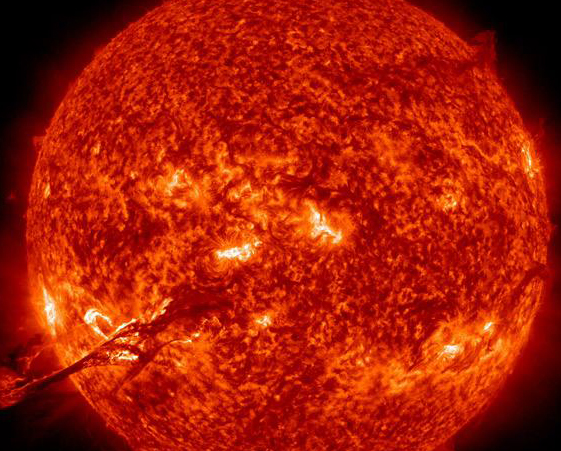µ IB | Measurements

10 quick questions

10 minutes maximum! (can you do it in 5?)

1. Which of these is a base quantity?

• A. current
• B. power
• C. energy
• D. acceleration

2. Which of these is a derived unit?

• A. amp
• B. kilogram
• C. joule
• D. second

3. Which of these numbers represents the prefix 'nano'?

• A. 10-3
• B. 10-6
• C. 10-9
• D. 10-12

4. 0.0004 joules can be written in millijoules (mJ) or microjoules (µJ). Which of these gives both conversions correctly?

A
 Energy in mJ Energy in µJ A 4 400 B 0.4 400 C 4 40 D 0.4 40

5. A power station has an output of 2.5 GW. This is

• A. 2 500 W
• B. 2 500 000 W
• C. 25 000 000 W
• D. 2 500 000 000 W6. A coulomb has base units of

• A. A s
• B. A s-1
• C. s A-1
• D. s-1 A-1

7. A rabbit is measured running a distance of 2.5 m in 3.45 seconds. The answer for the speed should be given as

• A. 2.5 ÷ 3.45 = 0.7246 m s-1
• B. 2.5 ÷ 3.45 = 0.724 m s-1
• C. 2.5 ÷ 3.45 = 0.72 m s-1
• D. 2.5 ÷ 3.45 = 0.7 m s-1

8. The unit [kg m s-2] is the same as a

• A. joule
• B. kilogram
• C. newton
• D. volt

9&10. These questions are about estimating. Try to do them WITHOUT a calculator!

A star is found to have :

• Mass = 2 x 1027 kg
• Radius = 1.1 x 108 m
• Volume = 1.8 x 1024 m39. The average density of the star is about

• A. 1057 kg m-3
• B. 1038 kg m-3
• C. 104 kg m-3
• D. 103 kg m-3

10. The surface area of the star is about

• A. 1017 m2
• B. 1015 m2
• C. 109 m2
• D. 108 m2